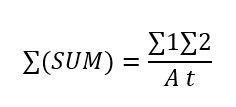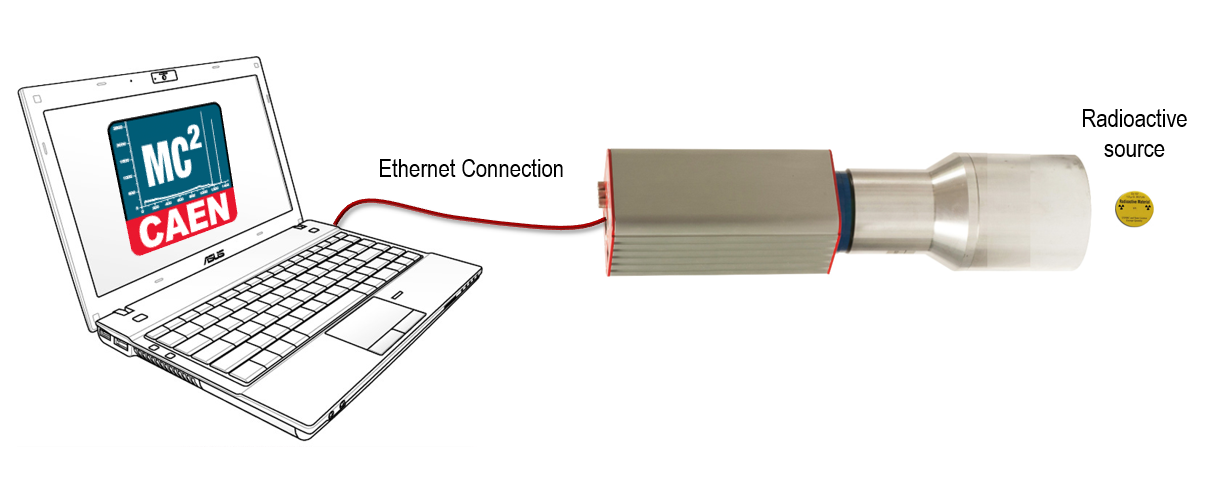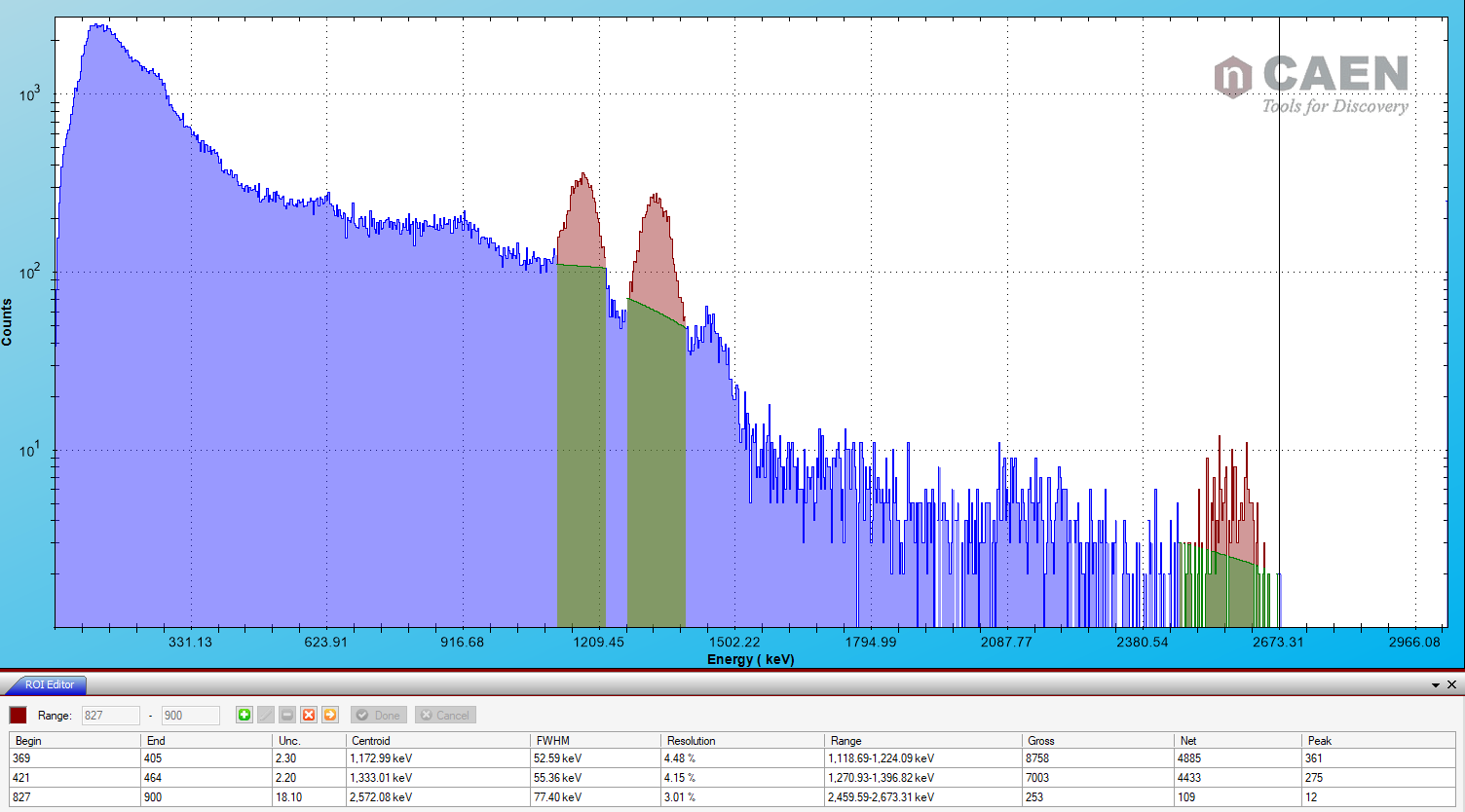Difficult Execution Time Data Analysis Radioactive SourcesYes Gamma

#### Equipment

SP5640Backpack Detector
Gamma Radioactive Source#### Purpose of the experiment

Determine the activity of a 60Co source from its gamma spectrum. Learn about the meaning of the sum peak, visible in the spectrum of some radioactive soruces.

#### Fundamentals

The 60Co spectrum presents two distinct gamma photopeak in its spectrum, respectively corresponding to photons γ1 and γ2 at 1.17 MeV and 1.33 MeV. For the purpose of this experiment, we can assume that each of these gamma rays are isotropically distributed. In other words, if γ1 departs in a particular direction, γ2 can go in any direction that it wishes. There is a certain probability that γ2 will go in the same direction as γ1. If this occurs the energies of γ1 and γ2 will be summed in the detector. Hence a sum peak will show up in the spectrum, at nearly 2.5 MeV.

We can estimate the activity of the source by calculating the counts under the two main peaks and under the sum peak, i.e. calculating their area ∑. For the case of 60Co, we have that the counts under the sum peak can be evaluated asWhere A is the activity of the source and t is the acquisition time.

Therefore, fitting the peaks with a gaussian and calculating their area, it is possible to estimate the activity of the 60Co source used to record the available spectrum.

#### Carrying out the experiment

The experiment can be performed by taking off the instrumentation from the backpack. To power ON γstream, press the ON/OFF button. Take care that the γstream internal battery is charged, otherwise use the external power system. Connect the Ethernet cable from γstream to the PC and configure the Ethernet network of your PC. Connect γstream to the MC2Analyzer software through Ethernet connection. Run the software and power ON the detector. Place the radioactive source close to the scintillator. Once the radioactive source is properly positioned, the spectrum can be recorded.#### Results

The student should verify that, after the spectrum calibration, the sum peak is nearly at 2.5 MeV. From the formula given above, using the live time in seconds, the student can estimate de activity of 60Co directly in Bq. A calculation made for a spectrum acquired in 100 seconds gives an activity of nearly 264 kBq.The 60Co complete spectrum acquired by MC2Analyzer software.